# 前言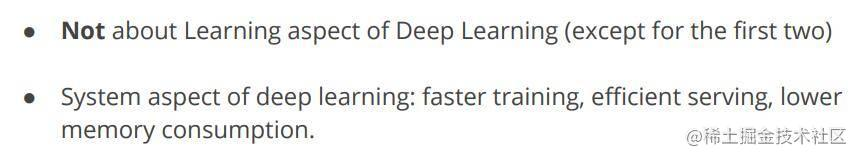## 什么是深度学习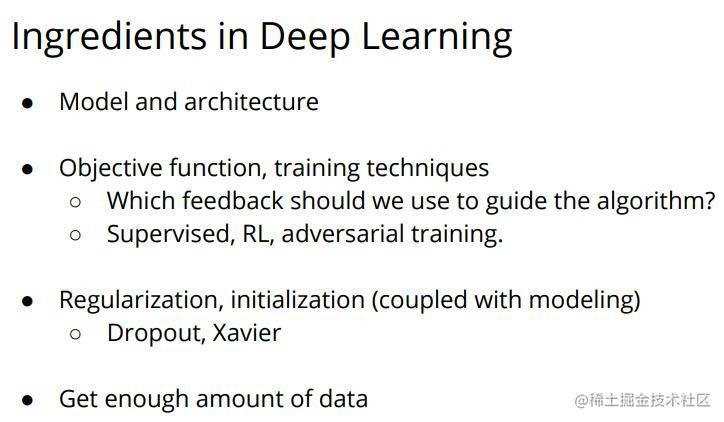• 模型的设计(最核心的地方，不同的任务设计的模型不同，使用的具体算法也不同)
• 目标函数，训练策略(用于训练模型权重参数的目标函数，也就是损失函数，以及一些训练的方法)
• 正则化和初始化(与训练过程中的模型权重信息有关系，加快训练的收敛速度，属于训练部分)
• 足够多的数据(机器学习亦或深度学习都是由数据驱动着了，没有数据的话就什么都不是)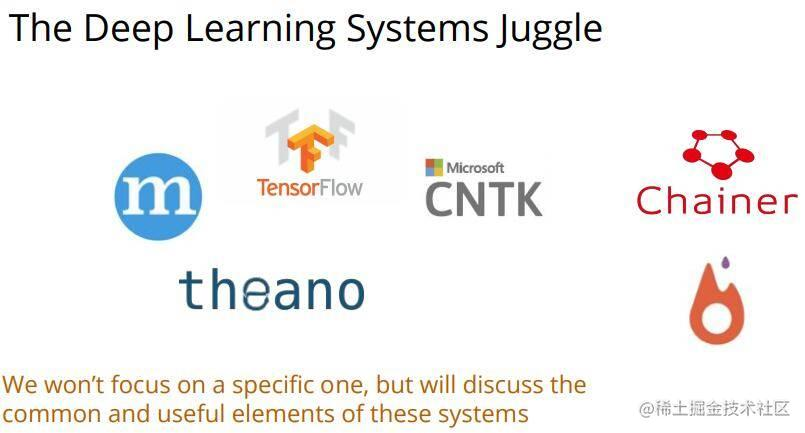• 用户API
• 系统组件
• 底层架构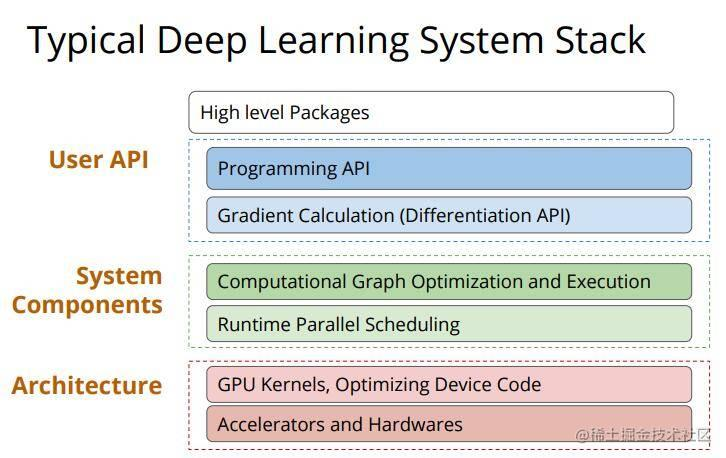## Logistic Regression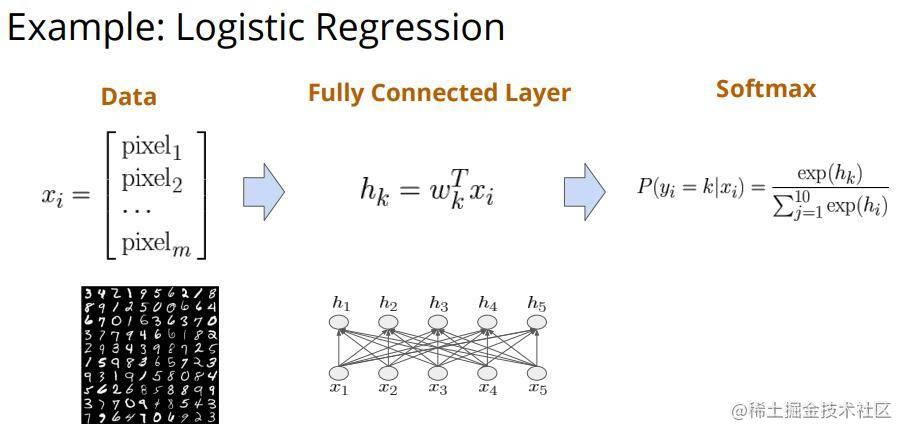• 前向计算：因为只有一个全连接层，所以我们的计算也很简单$h_{k}=w_{k}^{T} x_{i}$，其中$x_{i}$为输入的数据，$w_{k}^{T}$为权重，$h_{k}$为权重向量和数据向量点乘的结果。计算出来结果后我们使用softmax函数对其进行分类计算得到对应十个数字的概率向量(最后输出的向量包含10个元素，分别为每个数字的可能性)
• 反向求导：我们求出权重W关于极大似然损失的导数，这里我们人工写了出来，在右图第二部分
• 梯度更新：我们简单对权重W进行更新，更新值为学习率乘以W的梯度，即$w \leftarrow w-\eta \nabla_{w} L(w)$，也就是我们经常用的SGD

### 尝试使用TensorFlow like API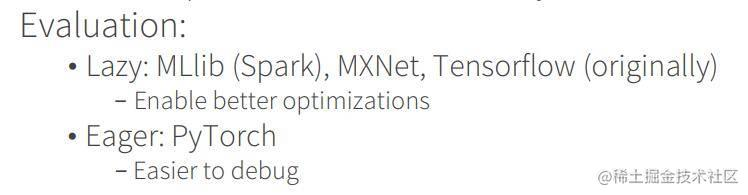• 输入x为float32类型的向量，[None,784]中None表示当前输入的batch-size未知
• W为权重信息，维度为[784,10]
• y为前向计算函数，利用算子函数嵌套定义了整个计算过程。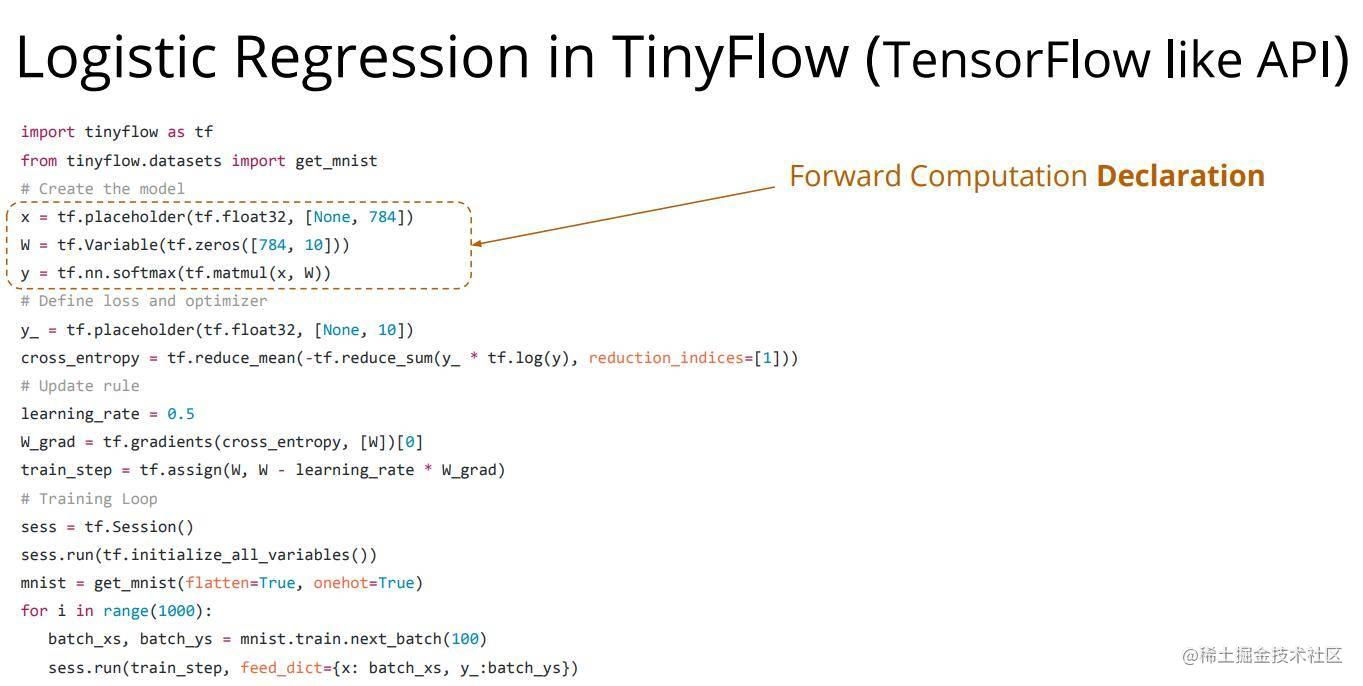cross_entropy = tf.reduce_mean(-tf.reduce_sum(y_*tf.log(y), reduction_indices=))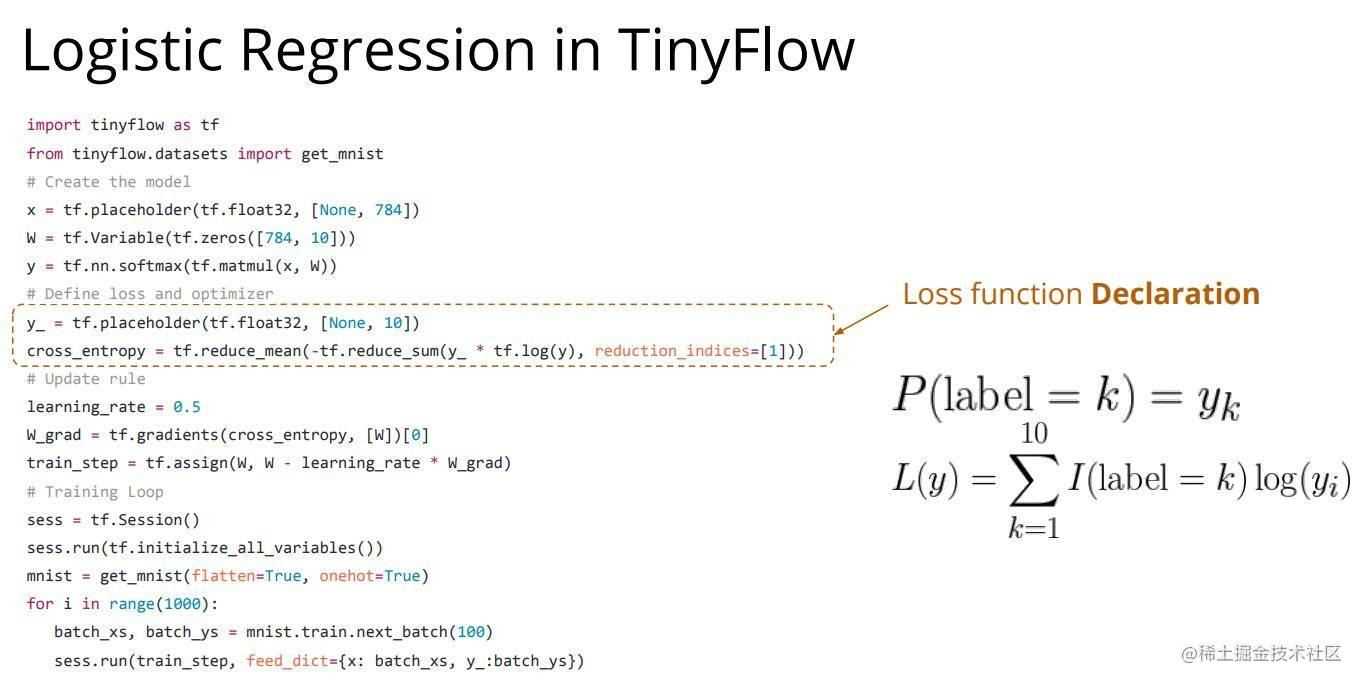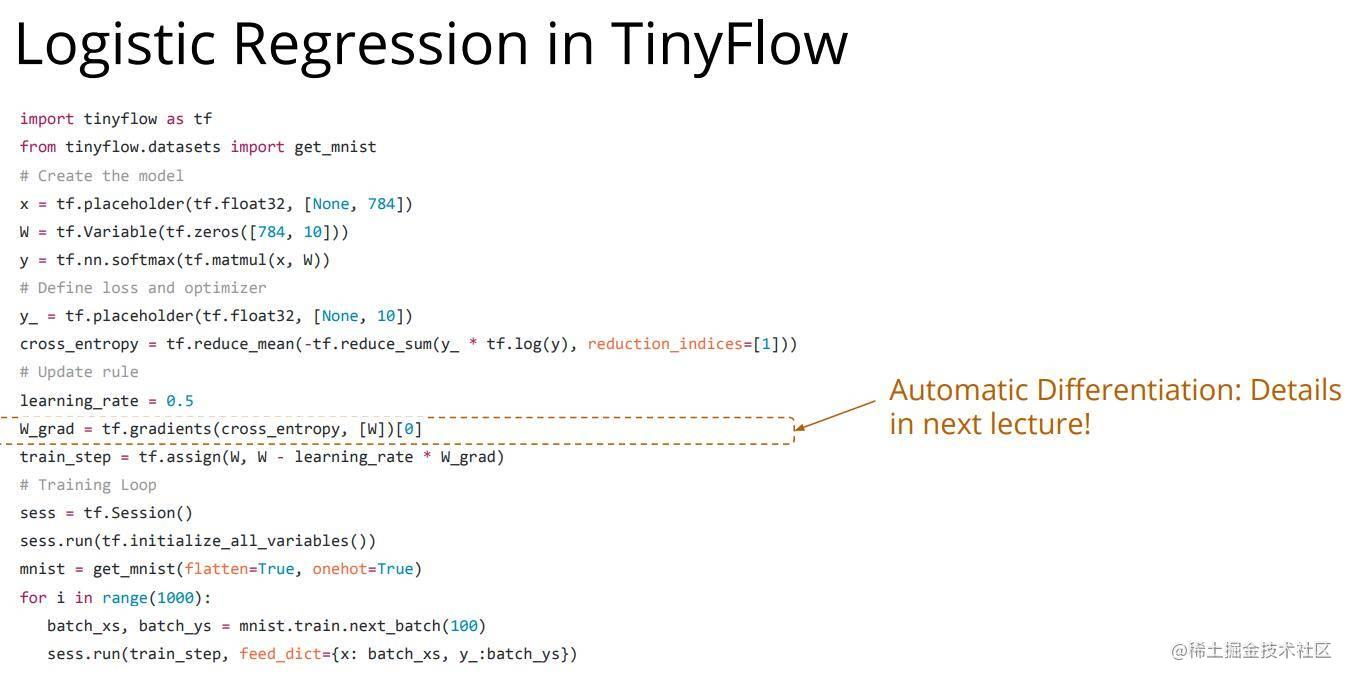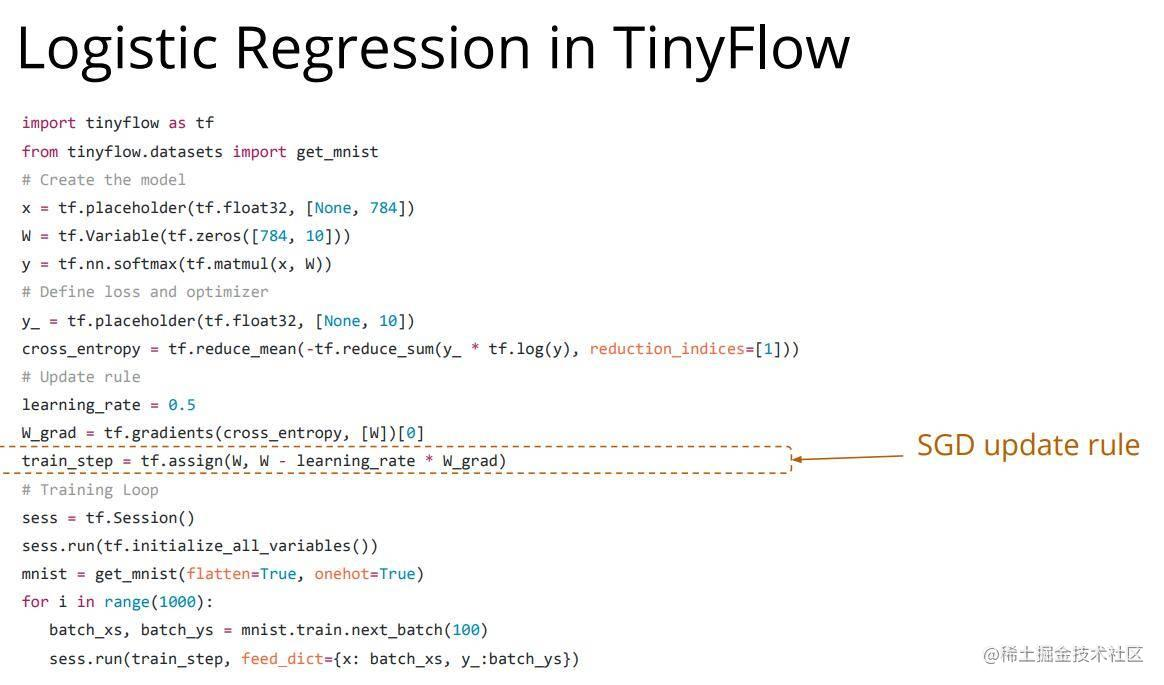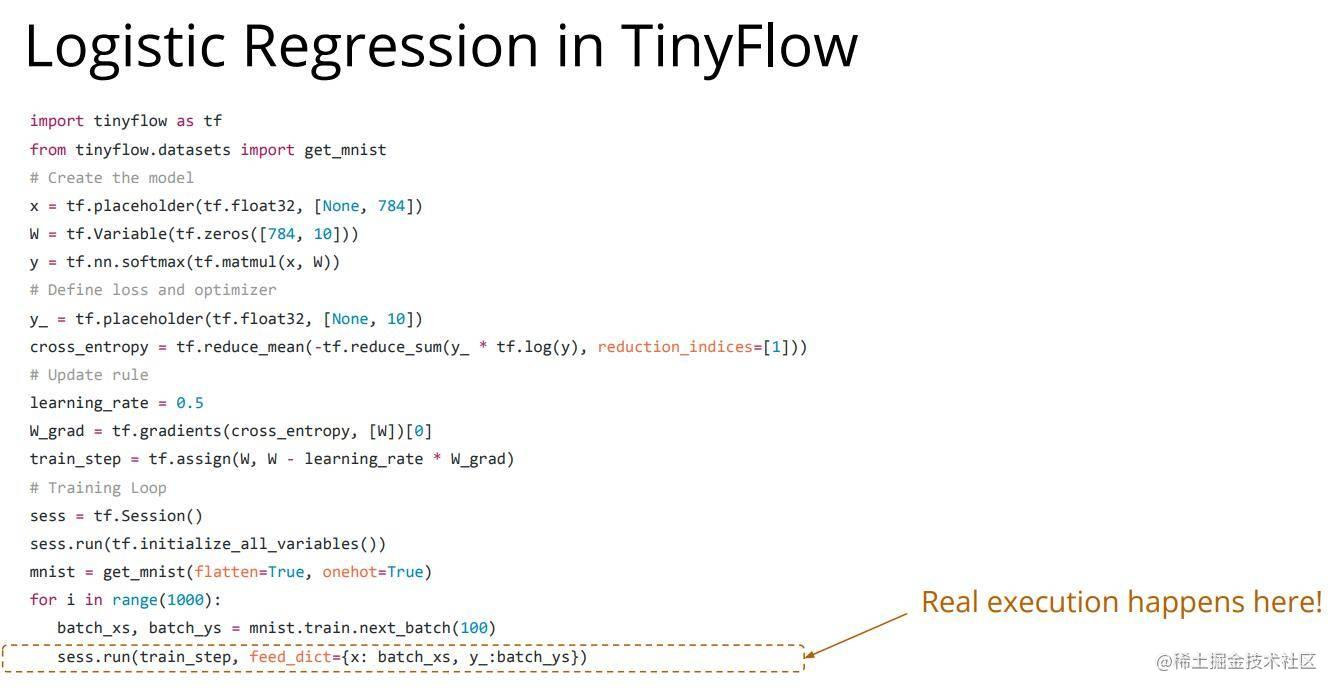### 计算图(Computation Graph)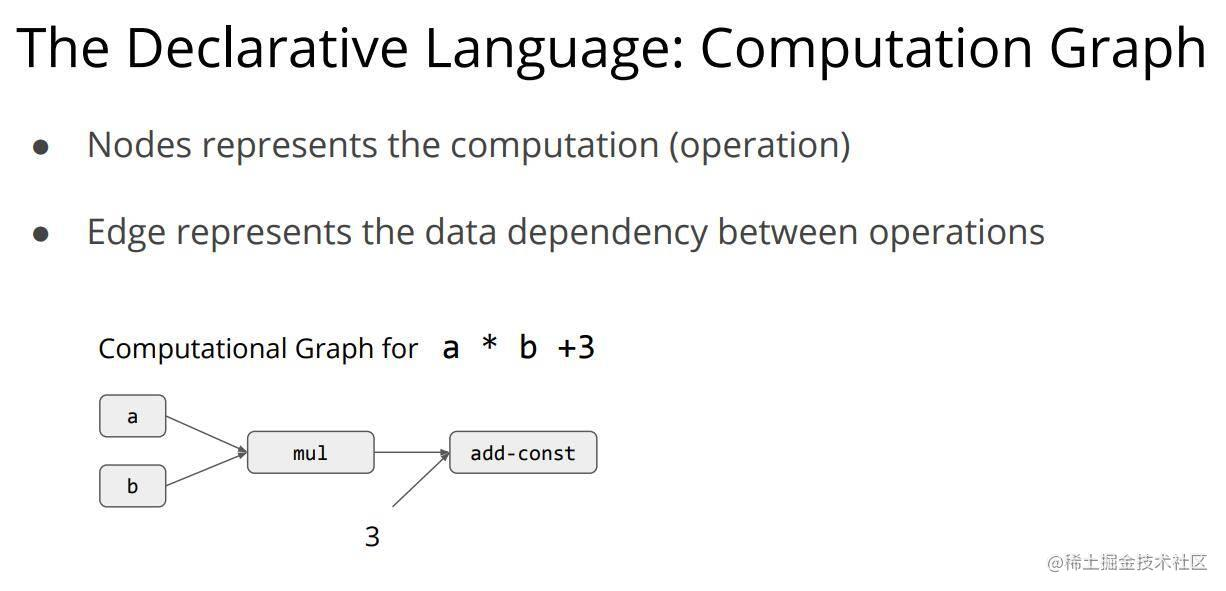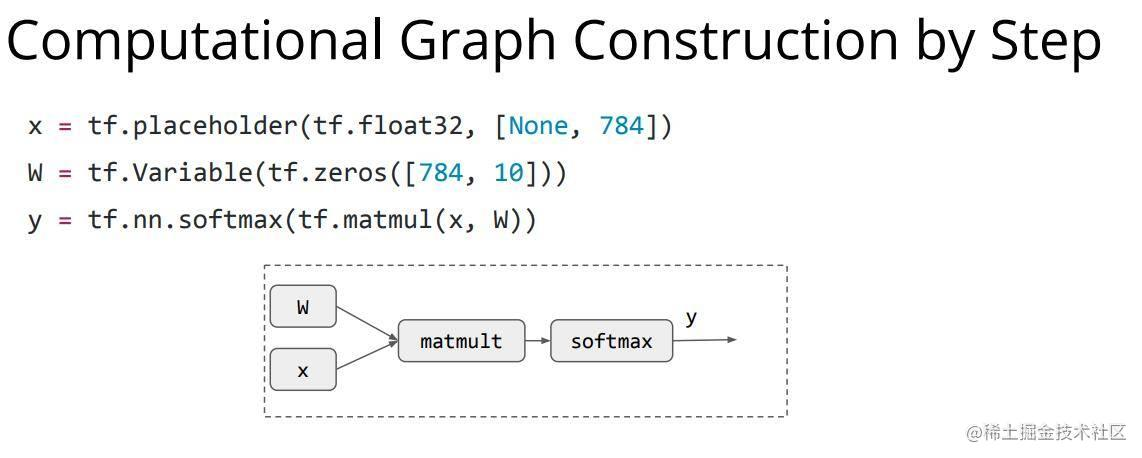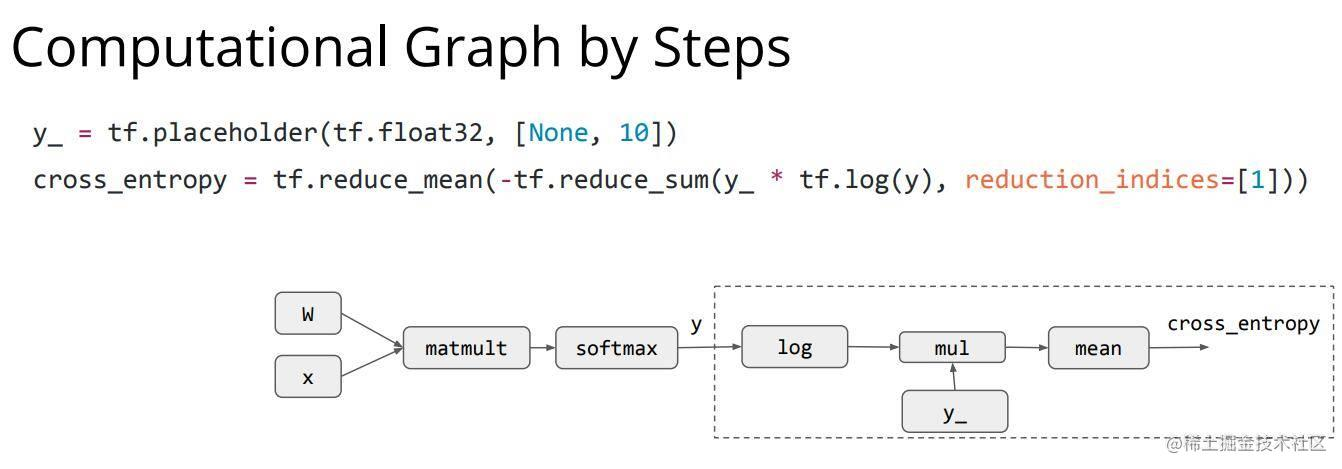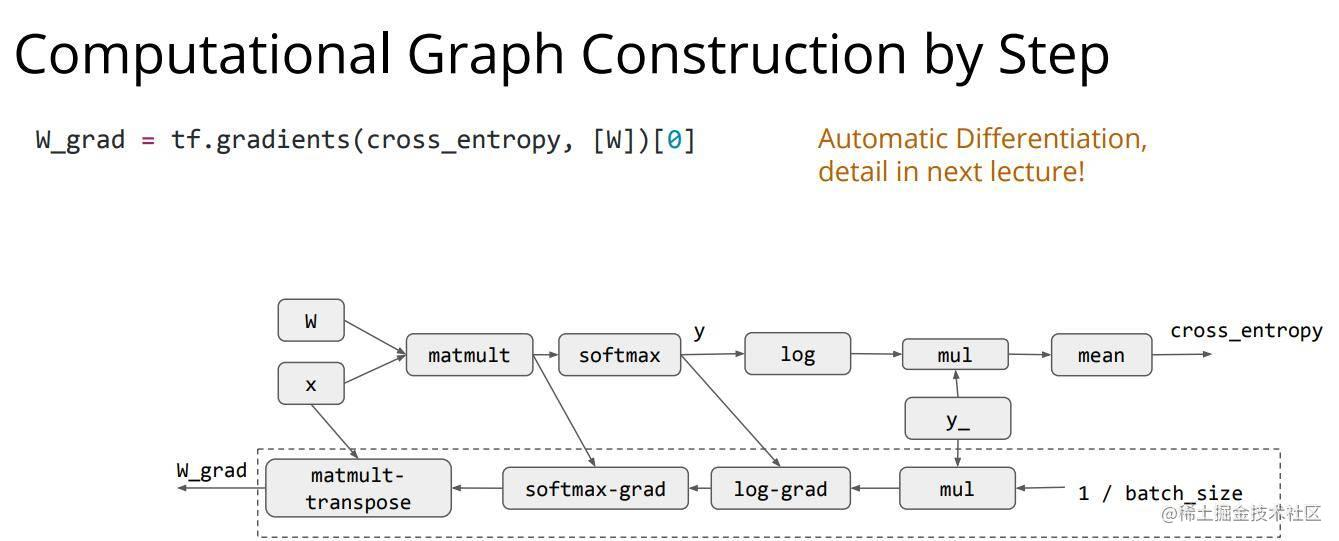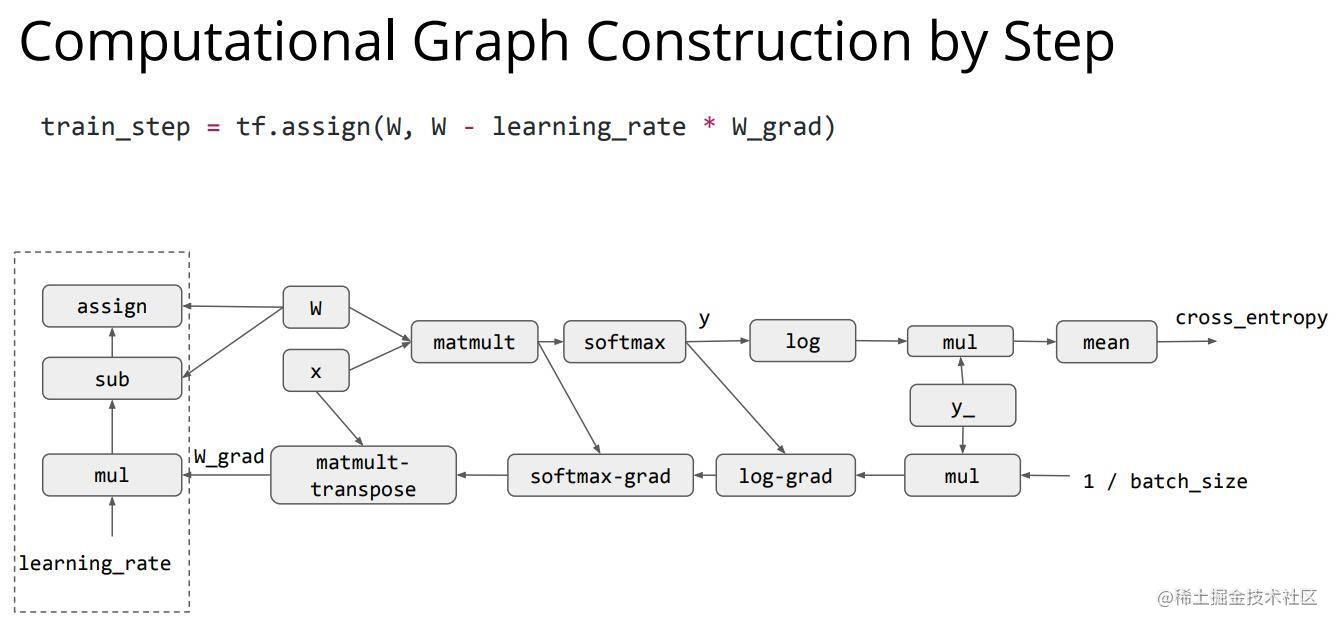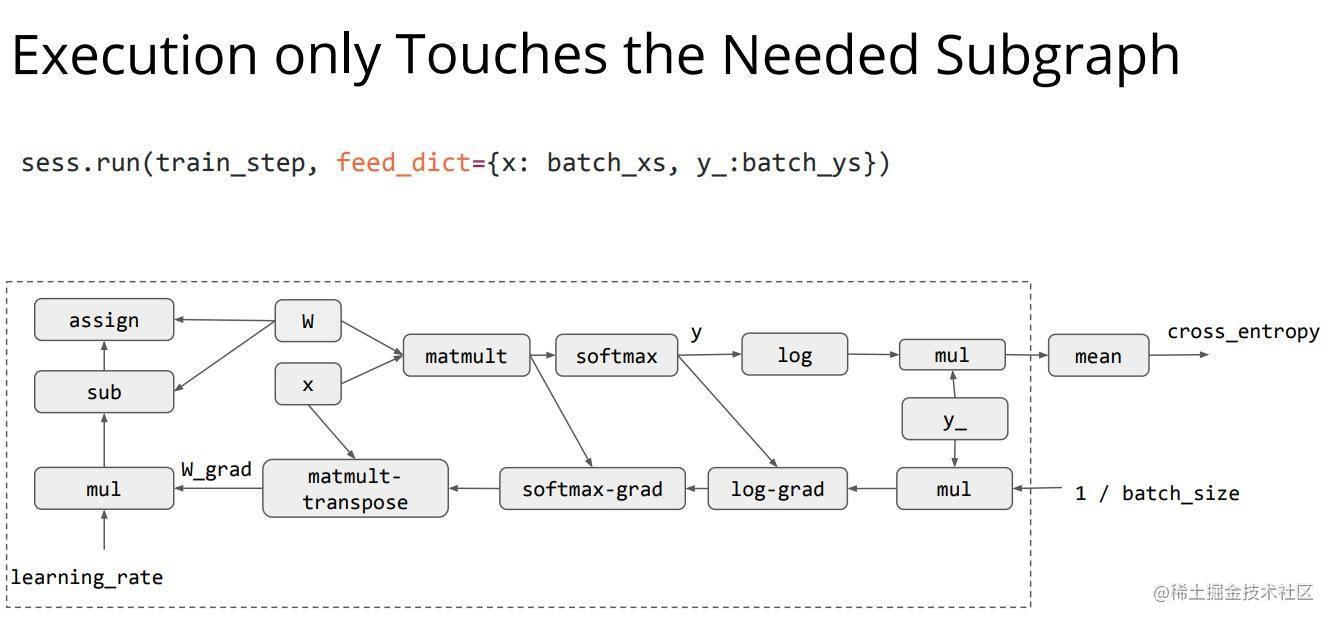### numpy 与 TF-program 的比较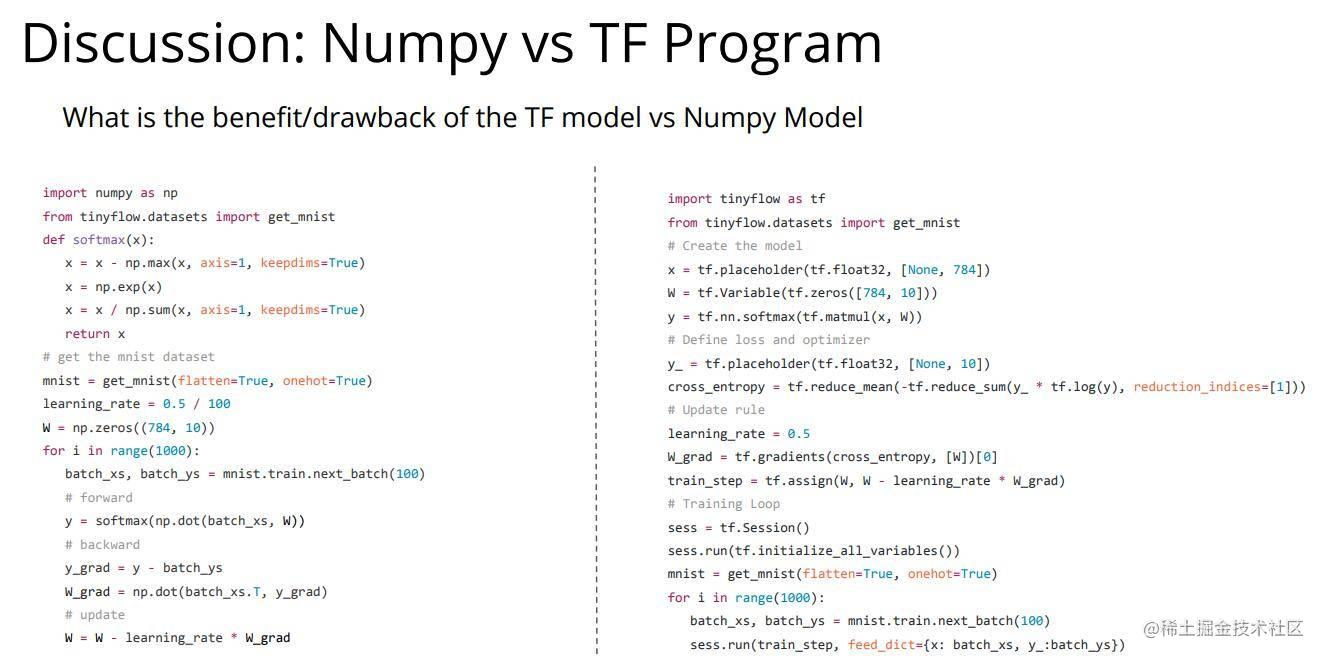## 未完待续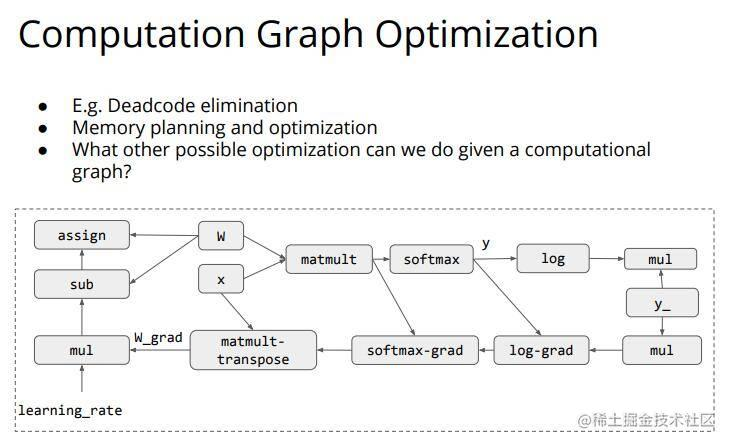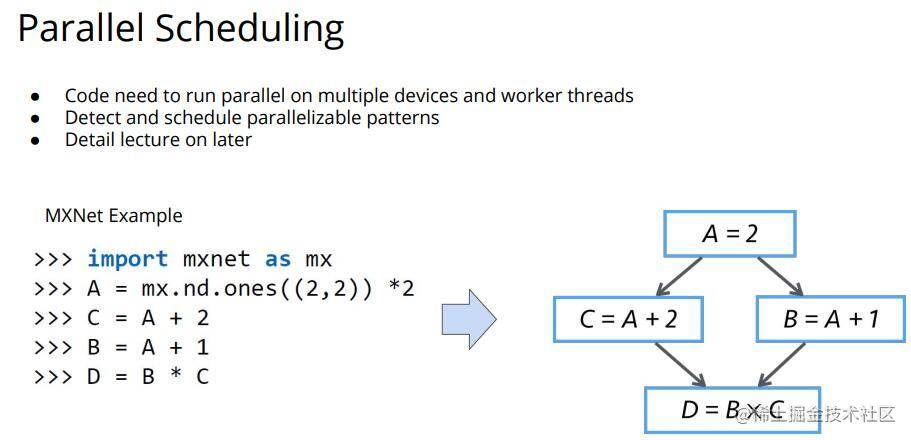# 撩我吧

• 如果你与我志同道合于此，老潘很愿意与你交流；
• 如果你喜欢老潘的内容，欢迎关注和支持。
• 如果你喜欢我的文章，希望点赞👍 收藏 📁 评论 💬 三连一下~